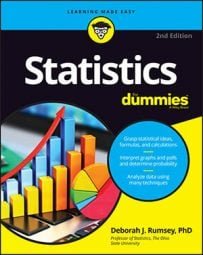##### Statistics For DummiesIf your data create a histogram that is not bell-shaped, you can use a set of statistics that is based on percentiles to describe the big picture of the data. Called the five-number summary, this method involves cutting the data into four equal pieces and reporting the resulting cutoff points that separate these pieces. These cutoff points are represented by a set of statistics that describe how the data are laid out.

A percentile is not a percent; a percentile is a number (or the average of two numbers) in the data set that marks a certain percentage of the way through the data.

The five numbers in a five-number summary are:

1. The minimum (smallest) value in the data set

2. The 25th percentile (also known as the first quartile, or Q1)

3. The median (50th percentile)

4. The 75th percentile (also known as the third quartile, or Q3)

5. The maximum (largest) value in the data set

For example, suppose you want to find the five-number summary of the following 25 (ordered) exam scores: 43, 54, 56, 61, 62, 66, 68, 69, 69, 70, 71, 72, 77, 78, 79, 85, 87, 88, 89, 93, 95, 96, 98, 99, 99.

To find the five-number summary, with n = 25, follow these steps:

1. Order all the values in the data set from smallest to largest.

Step 1 is done for this example because the data are ordered. In this example, 43 is the smallest number and 99 is the largest.

2. Multiply k percent times the total number of values, n.

This number is called the index. Since Q1 is the 25th percentile, multiply 0.25 x 25 = 6.25. For the median (50th percentile), multiply 0.5 x 25 = 12.5. For Q3 (the 75th percentile), multiply 0.75 x 25 = 18.75. Hence the indices for Q1, the median, and Q3 are 6.25, 12.5, and 18.75, respectively.

3. If the index from Step 2 is not a whole number, round it up to the nearest whole number and go to Step 4a. If your result from Step 2 is a whole number, go to Step 4b.

In this example case, the index for Q1 is not a whole number, so round it up from 6.25 to 7. Similarly, round the index for the median up from 12.5 to 13, and the index for Q3 up from 18.75 to 19. Then proceed to Step 4a.

4. 4a.Count the values in your data set from left to right (from the smallest to the largest value) until you reach the number indicated by Step 3. The corresponding value in your data set is the kth percentile.

Count from left to right in the data set until you reach the 7th value, 68; this is Q1. The 13th value on the list is 77, so that’s the median. The 19th value on the list is 89, so that’s Q3.

5. 4b.Count the values in your data set from left to right (smallest to largest) until you reach the number indicated by Step 2. The kth percentile is the average of that corresponding value in your data set and the value that directly follows it.

Step 4b doesn’t apply in this case, as none of the indices from Step 2 were whole numbers.

Putting it all together, the five-number summary for these 25 test scores is 43, 68, 77, 89, and 99.

The steps shown here demonstrate one way of calculating the median and quartiles of the five-number summary, but there are several other acceptable methods. Do not be too alarmed if your calculator or a friend gives you a set of values close to but different from what these steps would give.